## Tuesday, May 26, 2009

### The Schrödinger Equation

Update: A corrected and improved version of this post is now up: http://behindtheguesses.blogspot.com/2009/06/schrodinger-equation-corrections.html

notElon asked me to discuss, and to try and derive the Schrödinger equation, so I'll give it a shot. This derivation is partially based on Sakurai, with some differences.

A brief walk through classical mechanics
Say we have a function of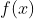and we want to translate it in space to a point. To do this, we'll find a ``space translation'' operator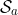which, when applied to, gives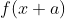. That is,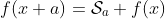(1)
We'll expandin a Taylor series: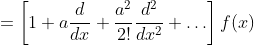(2)
which can be simplified using the series expansion of the exponential1 to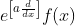(3)
from which we can conclude that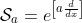(4)
If you do a similar thing with rotations around the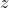-axis, you'll find that the rotation operator is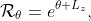(5)
where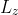is the-component of the angular momentum.

Comparing (4) and (5), we see that both have an exponential with a parameter (distance or angle) multiplied by something (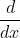or). We'll call the something the ``generator of the transformation.'' So, the generator of space translation isand the generator of rotation is. So, we'll write an arbitrary transformation operatorthrough a parameter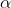as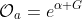(6)
where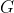is the generator of this particular transformation.2 See  for an example with Lorentz transformations.

From classical to quantum
In classical dynamics, the time derivative of a quantity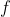is given by the Poisson bracket: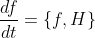(7)
where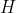is the classical Hamiltonian of the system and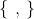is shorthand for a messy equation. In quantum mechanics this equation is replaced with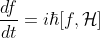(8)
where the square brackets signify a commutation relation and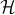is the quantum mechanical Hamiltonian. This holds true for any quantity, and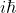is a number which commutes with everything, so we can argue that the quantum mechanical Hamiltonian operator is related to the classical Hamiltonian by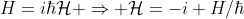(9)
specifically.

Additionally, we can extend from here that any quantum operator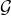is written in terms of its classical counterpartby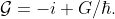(10)

So, using (4) the quantum mechanical space translation operator is given by(11)
and, using (5), the rotation operator by(12)
or, from (6) any arbitrary (unitary) transformation,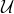, can be written as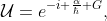(13)
whereis (an Hermitian operator and is) the classical generator of the transformation.

Time translation of a quantum state
Consider a quantum state at timedescribed by the wavefunction. To see how the state changes with time, we want to find a ``time-translation'' operator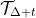which, when applied to the state, will give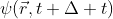. That is,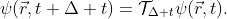(14)
From our previous discussion we know that if we know the classical generator of time translation we can writeusing (13). Well, classically, the generator of time translations is the Hamiltonian! So we can write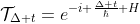(15)
and (14) becomes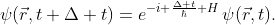(16)

This holds true for any time translation, so we'll consider a small time translation and expand (16) using a Taylor expansion3 dropping all quadratic and higher terms: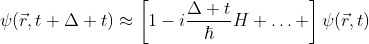(17)
Moving things around gives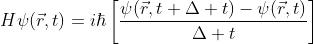(18)
In the limit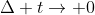the righthand side becomes a partial derivative giving the Schrödinger equation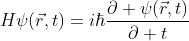(19)

For a system with conserved total energy, the classical Hamiltonian is the total energy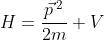(20)
which, making the substitution for quantum mechanical momentum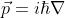and substituting into (19) gives the familiar differential equation form of the Schrödinger equation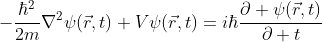(21)

References
 J.J. Sakurai. Modern Quantum Mechanics. Addison-Wesley, San Francisco, CA, revised edition, 1993.
 J.D. Jackson. Classical Electrodynamics. John Wiley & Sons, Inc., 3rd edition, 1998.
 L.D. Landau and E.M. Lifshitz. Mechanics. Pergamon Press, Oxford, UK.
 L.D. Landau and E.M. Lifshitz. Quantum Mechanics. Butterworth-Heinemann, Oxford, UK.
 H. Goldstein, C. Poole, and J. Safko. Classical Mechanics. Cambridge University Press, San Francisco, CA, 3rd edition, 2002.

1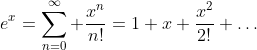2 There are other ways to do this, differing by factors ofin the definition of the generators and in the construction of the exponential, but I'm sticking with this one for now.
3 Kind of the reverse of how we got to this whole exponential notation in the first place...